# Approximate Integration

The following article is from The Great Soviet Encyclopedia (1979). It might be outdated or ideologically biased.

## Approximate Integration

the branch of numerical analysis devoted to the development and application of methods for the approximate evaluation of definite integrals.

Let y = f(x) be a continuous function on the closed interval [a, b] and let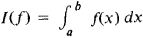If the values of an antiderivative F(x) of f(x) are known at x = a and x = b, then by the Newton-Leibniz formula (the fundamental theorem of the integral calculus)

I(f) = F(b) – F(a)

If F(d) and F(b) are not known, the value of I(f) must be found by other means. One way is to approximate the value of I(f) by means of quadrature formulas, that is, suitable linear combinations of a finite number of values of f(x) and its derivatives. A quadrature formula containing only values of the function f(x) takes the form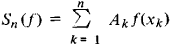The points xk, k = 1, 2, …, n, xk∈ [a, b], are called nodes and the coefficients Ak are called weights.

For every continuous function f(x), the value of I(f) can be computed by means of sums Sn (f) with arbitrary accuracy. The choice of quadrature formula is determined by the class Ω to which the given function f(x) belongs, by the method by which it is specified, and by the available computational resources. The error of the quadrature formula is the difference

Rn(f) = I(f) – Sn(f)

A quadrature formula contains 2n + 1 parameters that are independent of f(x): n, and Ak where k = 1, 2, …, n. The parameters are chosen so that for f ∈ Ω the error is acceptably small. The accuracy of a quadrature formula for f ∈ Ω is characterized by the quantity rn (Ω), defined as the least upper bound of ǀRn (f)ǀ on Ω: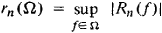Let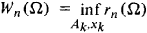A quadrature formula for which Wn(Ω) = rn (Ω) is said to be optimal on the class Ω. The weights and nodes in an optimal quadrature formula may be arbitrary or subject to certain constraints.

Quadrature formulas are divided into elementary and composite. Several methods have been developed for constructing elementary quadrature formulas. Let ωq((x), q = 0, 1, …, be a complete system of functions in Ω, and let any f(x) ∈ Ω be sufficiently well approximated by linear combinations of the initial functions ωq(x). Let Iq), q = 0, 1, 2, …, be computable exactly. For every n, the parameters of the quadrature formula can be determined from the requirement that

Iq) = Snq) q = 0, 1, …, m

for as large a value of m as possible. In the Newton-Cotes method, the nodes xk of the quadrature formula are chosen, and the weights Ak must be determined. In the Chebyshev method, certain constraints are imposed in advance on the weights Ak (for example, Ak = (ba)/n), and the nodes xk must be determined. In the Gauss method, both the weights Ak and the nodes xk must be determined. In the Markov method, j nodes, j < n, are fixed in advance, and the weights and remaining nodes must be determined. The accuracy of the quadrature formulas obtained by these methods increases substantially with a felicitous choice of the functions ωq(x).

The Newton-Cotes formulas are based on the system of functions wq = xq, where q= 0, 1, …. The nodes xk divide the integration interval into equal parts. Examples of these formulas are the rectangular rule, trapezoidal rule, and Simpson rule.

Integration on the interval [a, b] can be reduced to integration on the interval [–1, 1] by a change of variable. Thus the weights and nodes of elementary formulas on [a, b] can easily be determined if they are known for [–1, 1].

In the case of composite formulas, the initial integral can be represented in the form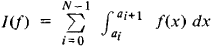Elementary quadrature formulas are used to compute the integrals on the intervals [ai, ai + 1].

In the Gauss formulas, m = 2n – 1. For a = –1 and b = 1, the nodes xk are the roots of the nth-degree Legendre polynominal Pn (x), and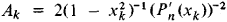The Chebyshev quadrature formula exists with Ak = l/n, l = ba, and xk ∈ [a, b] only for n = 1, …, 7, 9. In the Chebyshev formula m = n – 1. The use of equal weights minimizes the probability of error if the values of f(x) have independent random errors with the same variance.

In the computation of integrals of functions with period l, Gauss quadrature formulas are used most often. They are of the form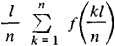There are quadrature formulas for the computation of integrals of the formwhere p(x) is a fixed weight function. It is selected so that for all f∈ Ω the functions f(x) are well approximated by linear combinations of the functions ωq(x).

For the approximate computation of indefinite integrals, the integrals are represented as definite integrals with a variable upper limit, and the formulas given above are used.

Tables of nodes, weights, and estimates of the error of quadrature formulas are given in special handbooks.

Quadrature formulas for the computation of multiple integrals are sometimes called cubature formulas. Multiple integrals can be computed as iterated integrals, using the quadrature formulas described above. Since the number of nodes increases substantially with the multiplicity, a number of special formulas have been developed for the computation of multiple integrals.

The calculation of integrals by computer is usually accomplished by means of standard programs. In the case of single integrals, standard programs with automatic calling sequences are most often used.

### REFERENCES

Krylov, V. I. Priblizhennoe vychislenie integralov, 2nd ed. Moscow, 1967.
Bakhvalov, N. S. Chislennye metody. Moscow, 1973.
Nikol’skii, S. M. Kvadraturnye formuly. Moscow, 1958.
Berezin, I. S., and N. P. Zhidkov. Metody vychisleniia, 3rd ed., part 1. Moscow, 1966.
Sobolev, S. L. Vvedenie v teoriiu kubaturnykh formul. Moscow, 1974.
Korobov, N. M. Teoretikochislovye metody v priblizhennom analize. Moscow, 1963.

V. I. LEBEDEV